# Exponent facts for kids

Kids Encyclopedia Facts

In mathematics, an exponent indicates how many copies of a number (known as the base) is multiplied together.

For example, in the number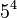$5^4$, 5 is the base and 4 is the exponent. This can be read as "5 to the power of 4". Therefore, in this example, four copies of 5 are multiplied together, which means that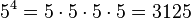$5^4 = 5\cdot5\cdot5\cdot5 = 3125$.

In general, given two numbers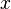$x$ and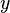$y$, the number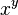$x^y$ can be read as "$x$ raised to the power of$y$" or "$x$ to the$y$th power", where$y$ is the exponent.

Bases raised to the power of two,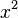$x^2$, can be read the base "squared", because the area of a square with a side length of x is$x^2$.

Similarly, bases raised to the power of three,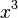$x^3$, can be called the base "cubed", because the area of a cube with a side length of x is$x^3$.

Exponents are used in algebra. Exponentiation is a way of shortening the process/equation of repeatedly multiplying many copies of a number.

An exponent is a number or symbol, placed above and to the right of the expression to which it applies, that indicates the number of times the expression is used as a factor. For example. the exponent x in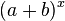$(a + b)^x$ indicates x copies of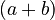$(a + b)$ are multiplied together.

There are some basic rules used in exponentiation

1. Product Rule: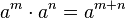$a^m \cdot a^n=a^{m+n}$

Proof - When multiplying a base term by two different exponents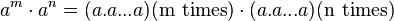$a^m \cdot a^n = (a . a ... a) (\text{m times})\cdot (a . a... a)(\text{n times})$, there are m number of a's and n number of a's.

Since exponentiation is simply repeated multiplication of a base term, we get that: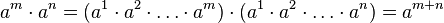$a^m \cdot a^n = (a^1 \cdot a^2 \cdot \dots \cdot a^m)\cdot(a^1 \cdot a^2 \cdot \dots \cdot a^n) = a^{m+n}$

therefore$a^m \cdot a^n = a^{m+n}$.

For example,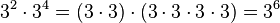$3^2 \cdot 3^4 = (3 \cdot 3) \cdot (3 \cdot 3 \cdot 3 \cdot 3) = 3^6$. This is true because at first, we had 2 threes times 4 threes which, when multiplied together, gives us 3 multiplied by itself 6 times, or 4+2 times.

2. Quotient Rule: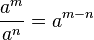$\frac{a^m}{a^n}=a^{m-n}$

Proof (when m > n) -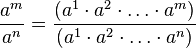$\frac{a^m}{a^n} = \frac{(a^1 \cdot a^2 \cdot \dots \cdot a^m)}{(a^1 \cdot a^2 \cdot \dots \cdot a^n)}$

By grouping the corresponding a’s, we get: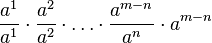$\frac{a^1}{a^1} \cdot \frac{a^2}{a^2} \cdot \dots \cdot \frac{a^{m-n}}{a^n} \cdot a^{m-n}$

Since corresponding a’s become 1, we are then left with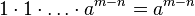$1 \cdot 1 \cdot \dots \cdot a^{m-n} = a^{m-n}$

therefore,$\frac{a^m}{a^n} = a^{m-n}$.

For example,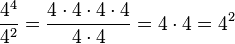$\frac{4^4}{4^2} = \frac {4 \cdot 4 \cdot4 \cdot4}{4 \cdot4} = 4 \cdot 4 = 4^2$. Notice that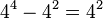$4^4 - 4^2 = 4^2$, which confirms the proof.

In fact, this property holds when m < n as well, but in that case, we get negative exponents instead. When m < n, the format of the problem becomes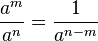$\frac{a^m}{a^n}= \frac{1}{a^{n-m}}$.

3. Zero Rule: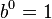$b^0 = 1$

Proof: we showed in #1 that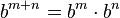$b^{m+n} =b^m \cdot b^n$.

This equation holds true for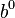$b^0$ as well, so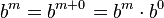$b^m = b^{m+0} = b^m \cdot b^0$

If this statement is true where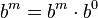$b^m = b^m \cdot b^0$, then$b^0$ must equal one. That is:$b^0 = 1$

## Negative exponents

A negative exponent is the reciprocal of a number with a positive exponent which can be mathematically represented as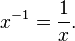$x^{-1}=\frac{1}{x}.$ In the same way that regular exponents are considered repeated multiplication, negative exponents can be considered repeated division.

Negative exponents can also be different from -1. In this case the negative exponent can be separated from the positive exponent, so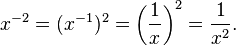$x^{-2}=(x^{-1})^2=\left(\frac{1}{x}\right)^{2}=\frac{1}{x^{2}}.$

The formula for a negative exponent in terms of a whole number exponent is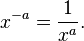$x^{-a}=\frac{1}{x^{a}}.$ There are more than one ways to prove this. The first proof involves a pattern form. It is not always a good idea to use patterns because in cases like exponents they may not be complete patterns for every case, but the proof still holds.

Proof #1: Why is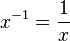$x^{-1} = \frac{1}{x}$ ?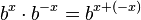$b^x\cdot b^{-x} = b^{x+(-x)}$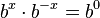$b^x\cdot b^{-x} = b^0$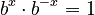$b^x\cdot b^{-x} = 1$ ***(see zero rule for proof of this!)

divide both sides by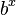$b^x$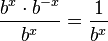$\frac{{b^{x}}\cdot{b^{-x}}}{b^{x}} = \frac{1}{b^{x}}$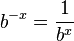$b^{-x} = \frac{1}{b^{x}}$

The second proof proves exactly the same thing but dives further into matching equalities

Proof #2:$x^{-1} = \frac{1}{x}$

everything in the next row is proved by the rules in the exponent section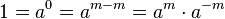$1= a^0 = a^{m-m}= a^m\cdot a^{-m}$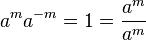$a^ma^{-m}= 1 = \frac {a^m}{a^m}$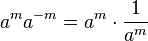$a^ma^{-m} = a^m \cdot \frac{1}{a^m}$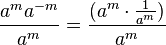$\frac {a^ma^{-m}}{a^m}= \frac {(a^m\cdot \frac {1}{a^m})}{a^m}$

therefore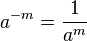$a^{-m} = \frac {1}{a^m}$

If we also have a multiplication, like in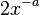$2x^{-a}$, it would be written as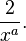$\frac{2}{x^{a}}.$

## Complex exponentiation

A complex number can also be taken to the power of another complex number. The relationship between imaginary numbers and trigonometry, which produces the multiplication formula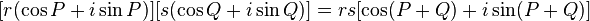$[r (\cos P + i \sin P)][s (\cos Q + i \sin Q)] = rs [\cos(P+Q) + i \sin(P+Q)]$

This formula basically states that the multiplication of complex numbers is related to addition of angles.

There are some relationships between functions and “infinite degree polynomials”, that are called series representations of the functions. The important series representations that we will focus on are: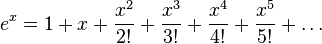$e^x = 1 + x + \frac{x^2}{2!} + \frac{x^3}{3!} + \frac{x^4}{4!} + \frac{x^5}{5!} + \dots$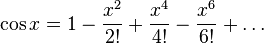$\cos x = 1 - \frac{x^2}{2!} + \frac{x^4}{4!} - \frac{x^6}{6!} + \dots$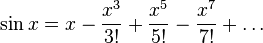$\sin x = x - \frac{x^3}{3!} + \frac{x^5}{5!} - \frac{x^7}{7!} + \dots$

From these representations, you should notice that the terms of the sine and cosine series combine, along with some sign changes, to make the exponential series. By introducing the number i into the equation, we get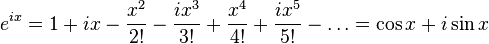$e^{ix} = 1 + ix - \frac{x^2}{2!} - \frac{ix^3}{3!} + \frac{x^4}{4!} + \frac{ix^5}{5!} - \dots = \cos x + i \sin x$

This result is often used as the beginning of the definition for complex number exponents.

There are some cases where we are raising other numbers to the power i. For example, we can use the following relationship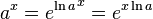$a^x = {e ^{\ln a}}^x = e^{x \ln a}$. Here using base 2 and exponent i, we get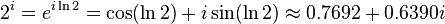$2^i = e^{i \ln 2} = \cos (\ln 2) + i \sin (\ln 2) \approx 0.7692 + 0.6390i$

When using base i and exponent i, we get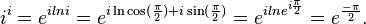$i^i = e^ {i ln i} = e^{i \ln {\cos (\frac{\pi}{2}) + i \sin (\frac{\pi}{2})}} = e^{i ln e ^{i \frac{\pi}{2}}} = e^{\frac{- \pi}{2}}.$

Now we can look at a general formula.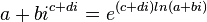$a + bi^{c + di} = e ^{(c+ di) ln (a + bi)}$

We can rewrite the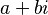$a + bi$ in the trigonometric form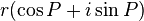$r (\cos P + i \sin P)$.

By the relationship we found between trigonometry and complex exponent, we can rewrite the equation in complex exponential form,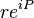$re^{iP}$.

Substituting this equation in the exponent in our original equation, we get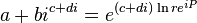$a + bi^{c + di} = e^{(c + di) \ln re^{iP}}$

This can be simplified to be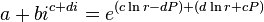$a + bi^{c + di} = e^{(c \ln r -dP) + (d \ln r + cP)}$

The real and imaginary parts of the exponent can be simplified separately to obtain to result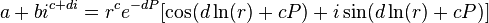$a + bi^{c + di} = r^c e^{-dP} [\cos (d \ln (r) + cP) +i \sin(d \ln (r) + cP)]$

Because there are many ways to describe the angle P, this formula can give many answers. If we allow the variable P to vary by multiples of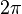$2 \pi$, we get the final result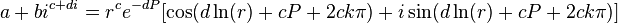$a + bi^{c + di} = r^c e^{-dP} [\cos (d \ln (r) + cP + 2 c k \pi) +i \sin(d \ln (r) + cP + 2 c k \pi)]$

## Images for kidsExponent Facts for Kids. Kiddle Encyclopedia.